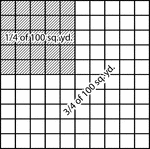### 1/4 of 100 Square Yards

A grid representing 100 square yards with 1/4 shaded.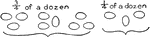### Dozen in Fourths

Visual of eggs showing 3/4 of a dozen and 1/4 of a dozen. 3x4=12, a dozen.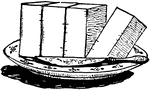### Fourths

A visual of a block cut into fourths.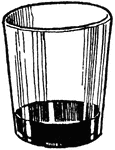### Glass Quarter Full

An illustration of a glass quarter full.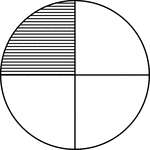### Fraction Pie Divided into Quarters

A circle divided into quarters with one quarter shaded.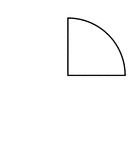### One Quarter of a Fraction Pie

One quarter of a circle.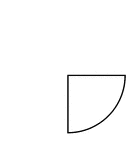### One Quarter of a Fraction Pie

One quarter of a circle.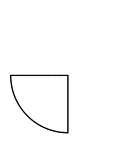### One Quarter of a Fraction Pie

One quarter of a circle.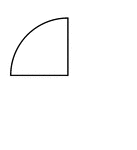### One Quarter of a Fraction Pie

One quarter of a circle.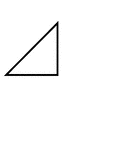### Fractions of 4-sided Polygon

1/4 of a 4 sided polygon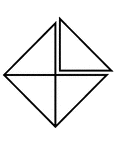### Fractions of 4-sided Polygon

4/4 of a 4 sided polygon with one piece detached